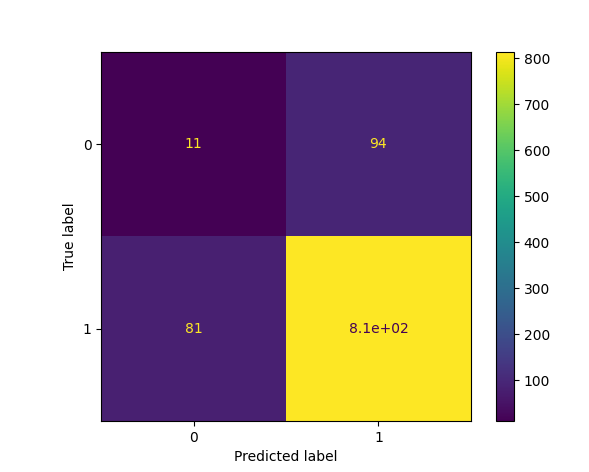## Python Tutorial

Python HOME Python Intro Python Get Started Python Syntax Python Comments Python Variables Python Data Types Python Numbers Python Casting Python Strings Python Booleans Python Operators Python Lists Python Tuples Python Sets Python Dictionaries Python If...Else Python While Loops Python For Loops Python Functions Python Lambda Python Arrays Python Classes/Objects Python Inheritance Python Iterators Python Polymorphism Python Scope Python Modules Python Dates Python Math Python JSON Python RegEx Python PIP Python Try...Except Python User Input Python String Formatting

## File Handling

Python File Handling Python Read Files Python Write/Create Files Python Delete Files

## Python Modules

NumPy Tutorial Pandas Tutorial SciPy Tutorial Django Tutorial

## Python Matplotlib

Matplotlib Intro Matplotlib Get Started Matplotlib Pyplot Matplotlib Plotting Matplotlib Markers Matplotlib Line Matplotlib Labels Matplotlib Grid Matplotlib Subplot Matplotlib Scatter Matplotlib Bars Matplotlib Histograms Matplotlib Pie Charts

## Machine Learning

Getting Started Mean Median Mode Standard Deviation Percentile Data Distribution Normal Data Distribution Scatter Plot Linear Regression Polynomial Regression Multiple Regression Scale Train/Test Decision Tree Confusion Matrix Hierarchical Clustering Logistic Regression Grid Search Categorical Data K-means Bootstrap Aggregation Cross Validation AUC - ROC Curve K-nearest neighbors

## Python MySQL

MySQL Get Started MySQL Create Database MySQL Create Table MySQL Insert MySQL Select MySQL Where MySQL Order By MySQL Delete MySQL Drop Table MySQL Update MySQL Limit MySQL Join

## Python MongoDB

MongoDB Get Started MongoDB Create Database MongoDB Create Collection MongoDB Insert MongoDB Find MongoDB Query MongoDB Sort MongoDB Delete MongoDB Drop Collection MongoDB Update MongoDB Limit

## Python Reference

Python Overview Python Built-in Functions Python String Methods Python List Methods Python Dictionary Methods Python Tuple Methods Python Set Methods Python File Methods Python Keywords Python Exceptions Python Glossary

## Module Reference

Random Module Requests Module Statistics Module Math Module cMath Module

## Python How To

Remove List Duplicates Reverse a String Add Two Numbers

## Python Examples

Python Examples Python Compiler Python Exercises Python Quiz Python Bootcamp Python Certificate

# Machine Learning - Confusion Matrix

On this page, W3schools.com collaborates with NYC Data Science Academy, to deliver digital training content to our students.

## What is a confusion matrix?

It is a table that is used in classification problems to assess where errors in the model were made.

The rows represent the actual classes the outcomes should have been. While the columns represent the predictions we have made. Using this table it is easy to see which predictions are wrong.

## Creating a Confusion Matrix

Confusion matrixes can be created by predictions made from a logistic regression.

For now we will generate actual and predicted values by utilizing NumPy:

``` import numpy ```

Next we will need to generate the numbers for "actual" and "predicted" values.

``` actual = numpy.random.binomial(1, 0.9, size = 1000) predicted = numpy.random.binomial(1, 0.9, size = 1000)```

In order to create the confusion matrix we need to import metrics from the sklearn module.

``` from sklearn import metrics```

Once metrics is imported we can use the confusion matrix function on our actual and predicted values.

``` confusion_matrix = metrics.confusion_matrix(actual, predicted)```

To create a more interpretable visual display we need to convert the table into a confusion matrix display.

``` cm_display = metrics.ConfusionMatrixDisplay(confusion_matrix = confusion_matrix, display_labels = [False, True])```

Vizualizing the display requires that we import pyplot from matplotlib.

``` import matplotlib.pyplot as plt```

Finally to display the plot we can use the functions plot() and show() from pyplot.

``` cm_display.plot() plt.show()```

See the whole example in action:

### Example

import matplotlib.pyplot as plt
import numpy
from sklearn import metrics

actual = numpy.random.binomial(1,.9,size = 1000)
predicted = numpy.random.binomial(1,.9,size = 1000)

confusion_matrix = metrics.confusion_matrix(actual, predicted)

cm_display = metrics.ConfusionMatrixDisplay(confusion_matrix = confusion_matrix, display_labels = [False, True])

cm_display.plot()
plt.show()

### ResultRun example »

## Results Explained

The Confusion Matrix created has four different quadrants:

True means that the values were accurately predicted, False means that there was an error or wrong prediction.

Now that we have made a Confusion Matrix, we can calculate different measures to quantify the quality of the model. First, lets look at Accuracy.

## Created Metrics

The matrix provides us with many useful metrics that help us to evaluate out classification model.

The different measures include: Accuracy, Precision, Sensitivity (Recall), Specificity, and the F-score, explained below.

## Accuracy

Accuracy measures how often the model is correct.

### How to Calculate

(True Positive + True Negative) / Total Predictions

### Example

Accuracy = metrics.accuracy_score(actual, predicted)
Run example »

## Precision

Of the positives predicted, what percentage is truly positive?

### How to Calculate

True Positive / (True Positive + False Positive)

Precision does not evaluate the correctly predicted negative cases:

### Example

Precision = metrics.precision_score(actual, predicted)
Run example »

## Sensitivity (Recall)

Of all the positive cases, what percentage are predicted positive?

Sensitivity (sometimes called Recall) measures how good the model is at predicting positives.

This means it looks at true positives and false negatives (which are positives that have been incorrectly predicted as negative).

### How to Calculate

True Positive / (True Positive + False Negative)

Sensitivity is good at understanding how well the model predicts something is positive:

### Example

Sensitivity_recall = metrics.recall_score(actual, predicted)
Run example »

## Specificity

How well the model is at prediciting negative results?

Specificity is similar to sensitivity, but looks at it from the persepctive of negative results.

### How to Calculate

True Negative / (True Negative + False Positive)

Since it is just the opposite of Recall, we use the recall_score function, taking the opposite position label:

### Example

Specificity = metrics.recall_score(actual, predicted, pos_label=0)
Run example »

## F-score

F-score is the "harmonic mean" of precision and sensitivity.

It considers both false positive and false negative cases and is good for imbalanced datasets.

### How to Calculate

2 * ((Precision * Sensitivity) / (Precision + Sensitivity))

This score does not take into consideration the True Negative values:

### Example

F1_score = metrics.f1_score(actual, predicted)
Run example »

All calulations in one:

### Example

#metrics
print({"Accuracy":Accuracy,"Precision":Precision,"Sensitivity_recall":Sensitivity_recall,"Specificity":Specificity,"F1_score":F1_score})
Run example »